# WBJEE Chemistry Test - 2

## 40 Questions MCQ Test WBJEE Sample Papers, Section Wise & Full Mock Tests | WBJEE Chemistry Test - 2

Description
Attempt WBJEE Chemistry Test - 2 | 40 questions in 60 minutes | Mock test for JEE preparation | Free important questions MCQ to study WBJEE Sample Papers, Section Wise & Full Mock Tests for JEE Exam | Download free PDF with solutions
QUESTION: 1

### Which of the following species can act both as Bronsted acid and base ? NH₂⁻ 2. HCO₃⁻ 3. NH₄⁺ 4. HSO₄⁻ Select the correct answer using the codes given below :

Solution: Bronsted lowry acid base definition:- Acid - which can donate H+ Base - which can accept H+ HCO3(-) acts as acid by donating H+ and becomes CO3(2-) , acts as base by accepting H+ and becomes H2CO3 similarly HSO4(-) becomes SO4(2-) and H2SO4. NH2(-) becomes NH3 and NH(2-)
QUESTION: 2

Solution:
QUESTION: 3

### Acetone and acetaldehyde are differentiated by

Solution:

Acetaldehyde gives silver mirror with Tollen's reagent
CH3CHO + 2[Ag(NH3)2] OH → CH3COONH4 + 2Ag + H2O + 3NH3
Acetone does not give this test

QUESTION: 4

Nitrates of all the metals are

Solution:
QUESTION: 5

Which of the following is not a preliminary test used to detect ions

Solution:
QUESTION: 6

The wavelength of light waves is inversely proportional to its

Solution:
QUESTION: 7

Which of the following alkyl group has the maximum + I effect?

Solution:
QUESTION: 8

The enzymes which convert glucose and fructose into ethyl alcohol is

Solution:
QUESTION: 9

Which of the the following has least bond energy?

Solution:
QUESTION: 10

The enthalpies of combustion of carbon and carbon monoxide are -393.5 and -283 KJ mol⁻¹ respectively .The enthalpy of formation of carbon monoxide per mol is

Solution:
QUESTION: 11

A glass bulb is filled with NO₂ gas and immersed in an ice bath at 0°C which becomes colourless after some time . This colourless gas will be

Solution:
QUESTION: 12

Units of rate constant for first and zero order reaction in terms of molarity (M) units are resectively

Solution:
QUESTION: 13

Given that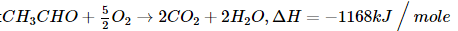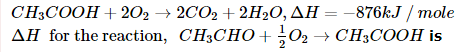Solution:
QUESTION: 14

The soaps are salts of higher fatty acids containing chains of

Solution:
QUESTION: 15

The systematic name of (CH₃)₂CH-COOH is

Solution:
QUESTION: 16

2,4 Dinitrophenyl hydrazine is an example for :

Solution: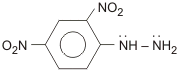is a monodentate ligand.

QUESTION: 17

The process associated with manufacture of sodium carbonate is known as

Solution:
QUESTION: 18

The density of gas at 27°C and 1 atm is d. Pressure remaining constant at which of the following temperatures will its density become 0.75 d ?

Solution:

At same pressure and same number of moles¸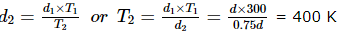QUESTION: 19

What is formed when boron is heated with concentrated H₂SO₄?

Solution:
QUESTION: 20

Which does not exist?

Solution:
QUESTION: 21

The P-P-P bond angle in white phosphorus is

Solution:
QUESTION: 22

Which one of the following substances is used in the laboratory for a fast drying of neutral gases?

Solution:
QUESTION: 23

Which of the following isomerism is not exhibited by alkanes?

Solution:
QUESTION: 24

Heaviest particle is

Solution: The heaviest particle of an atom is its neutron. It is due to its weight difference from others. It is 0.18% heavier than the proton which makes it the most heaviest.
QUESTION: 25

Which of the following on hydrolysis forms acetic acid?

Solution:
QUESTION: 26

Terylene is used for making

Solution:
QUESTION: 27

Which of the following is a polyamide molecule?

Solution:
QUESTION: 28

The oxidation number of Mn in KMnO₄ is

Solution:
QUESTION: 29

The crystal system of a compound with unit cell dimensions a=0.387, b=0.387 and c=0.0504 nm and α = β = 90°and γ = 120°is

Solution:
QUESTION: 30

In the Fischer projection formula, the representation of meso-butane-2,3-diol is :

Solution:
QUESTION: 31

Among the following, the least stable resonance structure is

Solution:

The resonance structure, in which the positive charges are present on the adjacent atoms, is expected to be least stable.

QUESTION: 32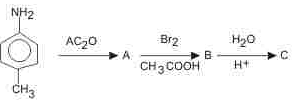The final product 'C' in the above reaction is

Solution:
QUESTION: 33

Which one of the following reactions of xenon compounds is not feasible?

Solution:
QUESTION: 34

Which of the following has an optical isomer?

Solution: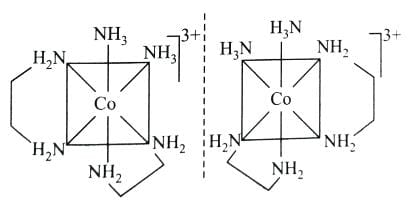QUESTION: 35

Toluic acid on reaction with Br₂ + Fe gives

Solution:
*Multiple options can be correct
QUESTION: 36

Which of the following sulphides undergoes self-reduction ?

Solution:
*Multiple options can be correct
QUESTION: 37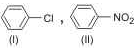Which is/are correct statement(s) about I and II ?

Solution:
QUESTION: 38

What is the best reagent to convert isopropyl alcohol to isopropyl bromide?​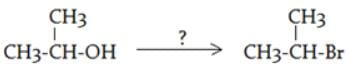Solution:

Thionyl bromide will react with secondary alcohols and give a reasonable percent yield of the bromide.

*Multiple options can be correct
QUESTION: 39

In which of the following cases entropy increases?

Solution: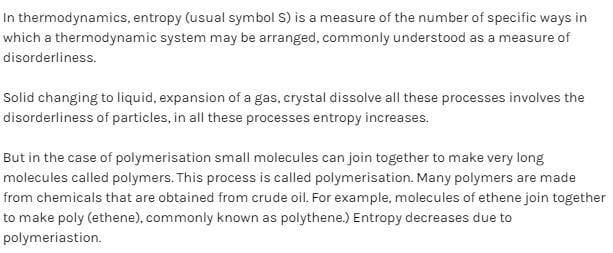*Multiple options can be correct
QUESTION: 40

Which of the following has an asymmetric carbon atom?

Solution:Use Code STAYHOME200 and get INR 200 additional OFF Use Coupon Code